Background
It is well known that many economic time series are difference stationary. In general, a regression involving the levels of these I(1) series will produce misleading results, with conventional Wald tests for coefficient significance spuriously showing a significant relationship between unrelated series (Phillips 1986).
Engle and Granger (1987) note that a linear combination of two or more I(1) series may be stationary, or I(0), in which case we say the series are cointegrated. Such a linear combination defines a cointegrating equation with cointegrating vector of weights characterizing the long-run relationship between the variables.
We will work with the standard triangular representation of a regression specification and assume the existence of a single cointegrating vector (Hansen 1992b, Phillips and Hansen 1990). Consider the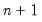dimensional time series vector process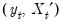, with cointegrating equation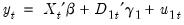(28.1)
where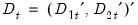are deterministic trend regressors and the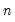stochastic regressors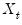are governed by the system of equations: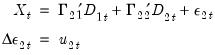(28.2)
The-vector of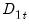regressors enter into both the cointegrating equation and the regressors equations, while the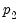-vector of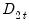are deterministic trend regressors which are included in the regressors equations but excluded from the cointegrating equation (if a non-trending regressor such as the constant is present, it is assumed to be an element ofso it is not in).
Following Hansen (1992b), we assume that the innovations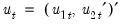are strictly stationary and ergodic with zero mean, contemporaneous covariance matrix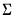, one-sided long-run covariance matrix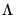, and covariance matrix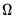, each of which we partition conformably with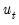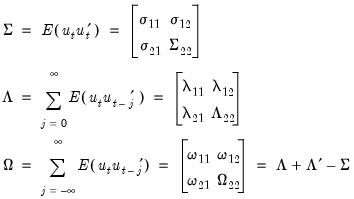(28.3)
In addition, we assume a ranklong-run covariance matrixwith non-singular sub-matrix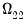. Taken together, the assumptions imply that the elements of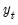andare I(1) and cointegrated but exclude both cointegration amongst the elements ofand multicointegration. Discussions of additional and in some cases alternate assumptions for this specification are provided by Phillips and Hansen (1990), Hansen (1992b), and Park (1992).
It is well-known that if the series are cointegrated, ordinary least squares estimation (static OLS) of the cointegrating vector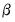in Equation (28.1) is consistent, converging at a faster rate than is standard (Hamilton 1994). One important shortcoming of static OLS (SOLS) is that the estimates have an asymptotic distribution that is generally non-Gaussian, exhibit asymptotic bias, asymmetry, and are a function of non-scalar nuisance parameters. Since conventional testing procedures are not valid unless modified substantially, SOLS is generally not recommended if one wishes to conduct inference on the cointegrating vector.
The problematic asymptotic distribution of SOLS arises due to the presence of long-run correlation between the cointegrating equation errors and regressor innovations and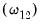, and cross-correlation between the cointegrating equation errors and the regressors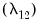. In the special case where theare strictly exogenous regressors so that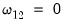and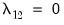, the bias, asymmetry, and dependence on non-scalar nuisance parameters vanish, and the SOLS estimator has a fully efficient asymptotic Gaussian mixture distribution which permits standard Wald testing using conventional limiting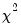-distributions.
Alternately, SOLS has an asymptotic Gaussian mixture distribution if the number of deterministic trends excluded from the cointegrating equationis no less than the number of stochastic regressors. Let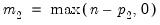represent the number of cointegrating regressors less the number of deterministic trend regressors excluded from the cointegrating equation. Then, roughly speaking, when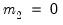, the deterministic trends in the regressors asymptotically dominate the stochastic trend components in the cointegrating equation.
While Park (1992) notes that these two cases are rather exceptional, they are relevant in motivating the construction of our three asymptotically efficient estimators and computation of critical values for residual-based cointegration tests. Notably, the fully efficient estimation methods supported by EViews involve transformations of the data or modifications of the cointegrating equation specification to mimic the strictly exogenouscase.